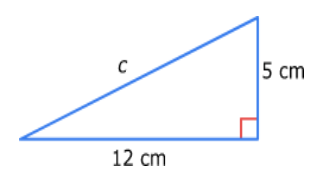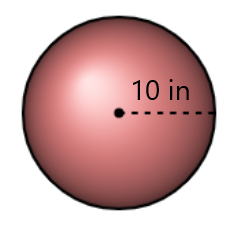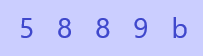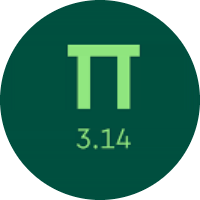# Practicing Various Mathematics Topics

In this quiz, You will get to know about various topics of Mathematics. You will find practice questions from all the topics like number theory, exponents, ratios, percentages, statistics, probability, etc.

Start Quiz

Evaluate.

(9⁹)¹²

9¹⁰⁸

9²¹

9²⁹

Subtract:

15/4 - 6/8 - 2/3 - 7/3

0

1/3

1

108 is what percent of 192?

50%

65.45%

56.25%

58.45%

Solve for t in the proportion.

28/63 = 16/t

t = 24

t = 36

t = 12

t = 28

A triangle has angle measurements of 40°, 56°, and 84°. What kind of triangle is it?

acute

obtuse

right

What is the length of the hypotenuse? If necessary, round to the nearest tenth25

13

17

9

A rectangle has a perimeter of 45.6 centimeters and a base of 12 centimeters. What is the height?

9.8 cm

12 cm

10.8 cm

15.8 cm

What is the volume of this sphere?

Use ​𝜋 ≈ 3.14 and round your answer to the nearest hundredth.4186.67

4356.33

3834.67

Type the missing number in this sequence:

4, 16, 64, ##256##, 1024, 4096

128 512 256

Evaluate the expression for s = 3.

4.s - 5 = ##7##

6 7 5

Which property of multiplication is shown?

6 × (1 × 2) = (6 × 1) × 2

commutative

associative

Which property of equality is shown below?

If: 92 = 32 − c

Then: 92 − d = 32 − c − d

Subtraction Property of Equality

Multiplication Property of Equality

Division Property of Equality

Solve for x.

-7(-5x + 10) + 1 = 10 + 6x + 8

x = 5

x = 3

x = 4

x = 2

Karen has the following data:If the range is 4, which number could b be?

8

10

12

9

Solve for a.

(-a/9) - 3 ≤ -4

a ≥ 5

a ≥ 9

a ≥ 6

A line passes through the points (0, 4) and (3, 10). What is its equation in slope-intercept form?

y = ##2##x + ##4##

3 -1 2 -2 4

Is (0, 3) a solution to the equation y = 4x?

yes

no

Use the function s = 3p - 6 to find the value of p when s = 3.

p = 3

p = 7

p = 0

p = 4

Which describes the system of equations below?

y = 2x + 9

y = (4/3)x + 5/9

consistent and independent

inconsistent

consistent and dependent

(7q + 5) + (5q + 8)

14q + 8

12q + 13

13q + 12

8q + 13

Quiz/Test Summary
Title: Practicing Various Mathematics Topics
Questions: 20
Contributed by: